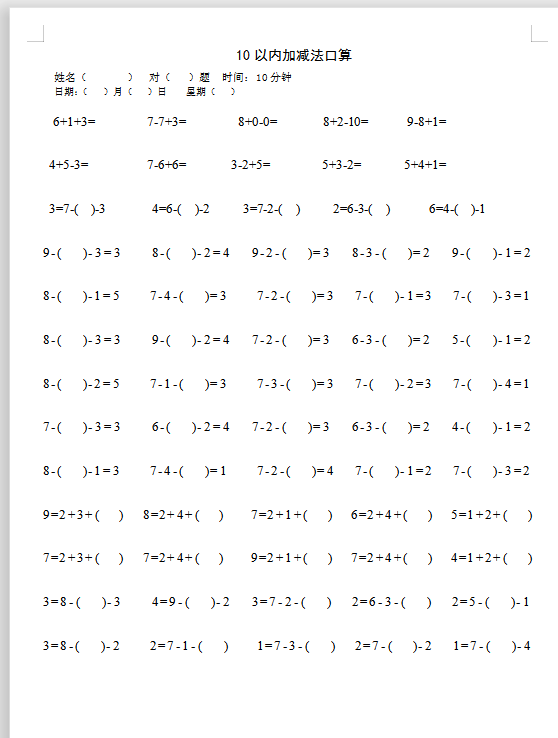10以内加减法口算

6+1+3=        7-7+3=        8+0-0=       8+2-10=      9-8+1=

4+5-3=         7-6+6=       3-2+5=        5+3-2=      5+4+1=

3=7-(  )-3       4=6-(  )-2     3=7-2-(  )     2=6-3-(  )      6=4-(  )-1

9-(  )-3=3   8-(  )-2=4  9-2-(  )=3  8-3-(  )=2  9-(  )-1=2